Similar triangles present one of the biggest tools students have to solve many geometry questions. And yet, whenever the opportunity arises, the students often fail to spot the similarity between two triangles. Sometime they spot the similarity but fail to apply the ratios correctly. Today we will start with some familiar cases in which we apply similarity of triangles and then move on to some tricky and difficult cases. We hope that after solving all these problems, you’ll not have any issue in spotting and solving similarity.

In practical scenario, we always prove that two triangles are similar by proving that their corresponding angles are equal. For example, look at the very simple figure below: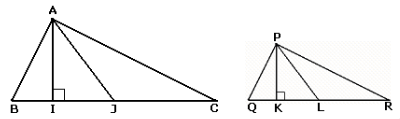If you can prove that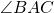=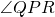and=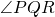then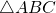and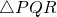are similar. In which case the ratios of the corresponding sides are equal. The best way to write the ratio of the sides is to write both the triangles in the order of the angle which are equal (for example here=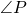,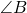=and=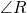so write ABC and PQR only) and then write the ratio by picking same corresponding points from the two written triangles. Therefore,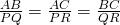Since the ratios of all the lengths (sides, perpendicular bisectors, medians, etc.) become the sameAlso, since area = length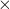length, the ratio of the areas of the two triangle is square of the ratio of the sides. That meansNow let’s apply these concepts to these problems. But first, let’s have a look at some common similar triangles scenarios which occur very often. These figures should automatically scream ‘similarity’ in our minds:Specially notice the second figure in the examples above, here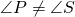but equal to. Therefore, writing the triangles in the order of angles which are equal,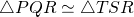Now let’s see some problems:

Problem 1: In the diagram given below,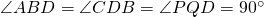If AB: CD = 3: 1, the ratio of CD: PQ is (CAT 2003- Leaked)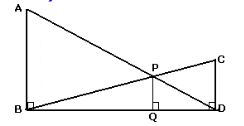1. 1: 0.69     2. 1: 0.75     3. 1: 0.72     4. None of these

Problem 2: In the figure, AB // DC. If the areas ofand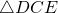are 4 and 9 respectively, find the area of1. 5     2. 6     3. 6.5     4. 9

Problem 3: In the square ABCD, AB is extended and E is a point on AB such that CE intersects AD at F and BD at G. The length of FG and GC are 3 and 5 units, respectively. What is the length of EF?1. 4     2. 5     3.4.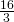Problem 4: Consider the triangle ABC shown in the following figure where BC = 12 cm, DB = 9 cm, CD = 6 cm and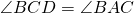. What is the ratio of the perimeter of the triangle ADC to that of the triangle BDC? (CAT 2005)
1.2.3.4.Problem 5: Given right triangle ABC, AD = DB, DE is perpendicular to AB, AB = 20 and AC = 12, find the area of the quadrilateral ADEC.1. 58.5     2. 56     3. 45     4. 37.5

Problem 6: PQRS is a rectangle. Diagonals PS and RQ intersect at point T. A perpendicular TU is drawn on PQ from T. U and R are joined. UR and PS intersect at V and a perpendicular VW is drawn on PQ. Now WR is drawn, intersecting PT at X and a perpendicular XY is drawn on PQ. If PQ = 90 units, find the length of PY.1. 40     2. 22.5     3. 20     4. 15

Problem 7: In the figure given below, O is a point inside the triangle PQR. Line segments ST, UV, XW are drawn through O, parallel to the sides PR, RQ and PQ respectively. The areas if three triangles SOV, WOU and TOX are 9, 25 and 36 respectively. What is the area of triangle PQR?1. 148     2. 169     3. 196     4. 200

Let’s check out a problem where you cannot see similarity at first sight.

Problem 8: Both right triangle ABC and isosceles triangle BCD, have height 5 cm from base BC = 12 cm. Find area of triangle BEC.